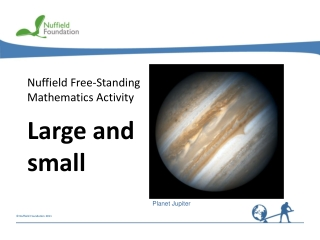DownloadDownload PresentationNuffield Free-Standing Mathematics Activity

# Nuffield Free-Standing Mathematics Activity

Download Presentation## Nuffield Free-Standing Mathematics Activity

- - - - - - - - - - - - - - - - - - - - - - - - - - - E N D - - - - - - - - - - - - - - - - - - - - - - - - - - -
##### Presentation Transcript

1. Nuffield Free-Standing Mathematics Activity Large and small Planet Jupiter

2. Large and small Jupiter’s surface area = 4r2 where r = 71 492 000 metres Think about…how to work out Jupiter’s surface area Image by STScI for NASA Atomic particles The mass of a proton is 0.000 000 000 000 000 000 000 001 673 gThis is 1836 times as heavy as an electron Think about…how to work out the mass of an electronor how many protons there are in 1kg Image by NASA/Goddard Space Flight Center Scientific Visualization Studio This activity shows the best way to work with very large and very small numbers like these.

3. = = = Powers of 10 103 101 10–2 10–3 102 100 10–1 1000 10 1 100 Think of a negative in the power as “1 over”. Any number to the power 0 is equal to 1. Other examples = 10  10  10  10  10  10 106 = 1 000 000 10–9

4. Standard form - a useful way of writing very large andvery small numbers Examples Mass of the Earth 6 000 000 000 000 000 000 000 000 kilograms = 6  1024 kilograms Think about…how to convert from one form to the other Distance from the Sun to Pluto 5 900 000 000 000 metres = 5.9  1012 metres

5. Examples The time between collisions of molecules in a gas 0.000 000 0002 seconds = 2  10–10 metres Think about…how to convert from one form to the other Mass of an atom of gold 0.000 000 000 000 000 000 0003 grams = 3 10–22 grams The wavelength of blue light 0.000 000 72 metres = 7.2  10–7 metres

6. Try these 9.25  10–4 4  106 7.3  105 3.681  10–7 2.4  1016 9.58  10–23 Standard form a 10n where a is between 1 and 10 and n is a positive or negative integer = 0.000 925 =4 000 000 =730 000 = 0.000 000 3681 =24 000 000 000 000 000 =0.000 000 000 000 000 000 000 0958

7. Examples 4.5  103 7.1  10–5 Calculating in standard form Use the EXP (or EE or  10x) key on a calculator Press4.5 EXP 3 Press7.1 EXP (-) 5 When you write down answers, remember the  10

8. Try these (4  10–3) +(8  10–5) (7  105) – (9  104) (8  109)  (2  106) (4  103)(2  106) Calculating in standard form =4.08  10–3 or0.004 08 =6.1  105 or610 000 =8  109 or 8 000 000 000 = 4  103 or 4000

9. Very large and very small Light split into its different colours by a prism

10. Large and small At the end of the activity Can you explain why: a 2.42  104 = 24 200 b 2.42  10–4 = 0.000 242 c 0.000 056 = 5.6  10–5 d 1 250 000 000 = 1.25  109 How do you enter a number like 5.6  10–5 into your calculator? What are the advantages of working in standard form? Are there any disadvantages?